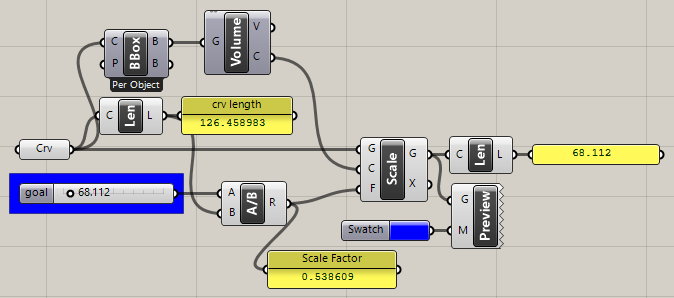# Gh python while Loop code

Hi , I am trying to create a small script to scale a curve till the curve length reaches a limit. I tried writing a gh python code, I am not able to understand Coerce 3d Point issue . Kindly help me with that Code . this is the code I have written .

import rhinoscriptsyntax as rs

# create a while loop for scaling a curve till it reaches the limit .

#rs.ScaleObject(
#print cpt
#newcpt = rs.coerce3dpoint(cpt)
#print newcpt

def crv_offset(crv1,lngthlmt):
new_crvlist =
if rs.IsCurveClosed(crv1)is False : return
if lngthlmt is None : return
cpt = rs.CurveAreaCentroid(curve)
while True:
if rs.CurveLength(crv1)<=lngthlmt:break
crv1 = rs.ScaleObject(crv1,(cpt,cpt,c[t),(Scale,Scale,Scale))
new_crvlist.append(crv1)
return new_crvlist

a = crv_offset(curve,limit)

I don’t know python, but this seems a risk:

Also the length of a curve is directly proportional to its size.
You can scale uniformly a curve by TargetLength / CurveLength and it will reach target length in 1 step, no loop needed.

Can you attach your current .gh with python code?

2 Likes

Wow, I didn’t know that. How foolish of me to do this crude approximation in Anemone.

scale_curve_2020Sep5a.gh (13.7 KB)

So much simpler and more accurate! Good to know, thanks.scale_curve_2020Sep5b.gh (10.3 KB)

2 Likes

Welcome @sharath.smith32,

If you’re still looking for a Pythonic recipe, this might do the trick!

Instead of using rhinoscriptsyntax, like you do, I use the API, which is a better fit for GHPython, and you don’t have to deal with coercing issues. These mostly stem from Grasshopper expecting geometry, but rhinoscriptsyntax mostly returning GUIDs, which are object IDs of sorts.

``````import Rhino.Geometry as rg

def scale_curve(curve, max_growth, step=0.01):

if not curve.IsClosed:
return

crv_length = curve.GetLength()
max_length = crv_length + crv_length / 100 * max_growth
if crv_length >= max_length:
return [curve]

new_curves = []
while crv_length < max_length:
if step == 0:
break
curve.Scale(1.0 + step)
new_curves.append(curve.DuplicateCurve())
crv_length = curve.GetLength()

return new_curves

if __name__ == "__main__":
a = scale_curve(Curve, 15.0, Step)
``````

I basically recreated your process, but integrated some exit strategies for the `while` loop.
Generally, these must be pretty watertight, otherwise you’ll end up with an infinite loop that will crash Rhino.
I compute the maximum curve length from a growth percentage, instead of using a static maximum length. This way you don’t have to check the initial curve length each time to define a higher value as maximum length. This is especially practical, if you want to apply the scaling to multiple curves, instead of just one!

scale_curve.gh (10.9 KB)

4 Likes